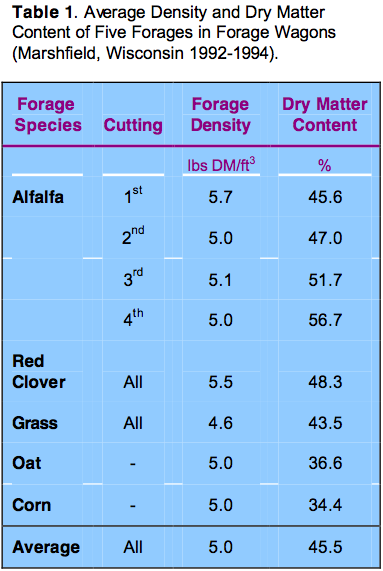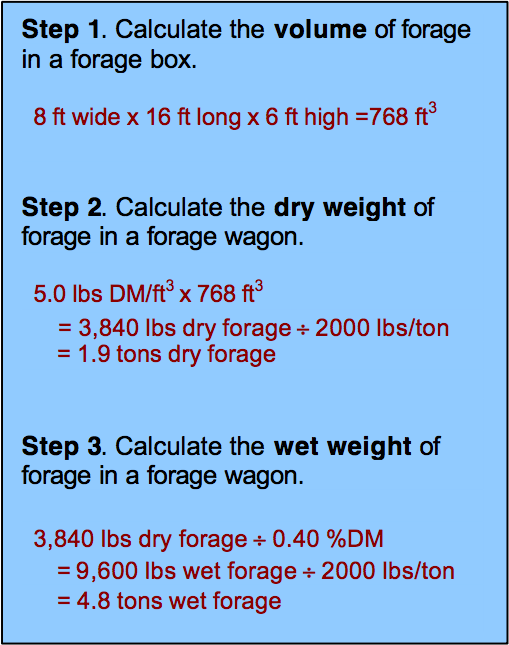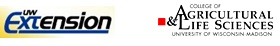# Estimating the Weight of Forage in a Forage Wagon

by Daniel W. Wiersma and Brian J Holmes

### Introduction

Most dairy farmers do not have a drive-over scale available on their farms. Frequently it would be useful to know the weight of forage harvested from a field. Measuring alfalfa or corn silage yield is necessary to adjust management practices, to maintain crop inventories, and for crop reporting purposes. Sometimes growers sell hay from a field and require an estimate of the quantity removed or sold.

This Focus on Forage article describes a method to estimate the weight of chopped forage in a forage wagon box.

### Wisconsin Forage Wagon Density StudyResearchers at the Marshfield Agricultural Research Station conducted a study to estimate the weight of loaded forage boxes. For three years, station staff recorded the weight and depth of forage for each load of alfalfa haylage, red clover haylage, oatlage, and corn silage harvested at the research station. In addition, forage samples were taken from each load to measure moisture content. Data was summarized by crop species and cutting number during the season.

Table 1 shows the average silage density of each forage species. Averaged over all species and cuttings, the density of forage in a wagon was 5.0 pounds of dry forage per cubic foot (lbs DM/ft³). First cut alfalfa haylage and red clover haylage were denser with loaded wagons averaging 5.6 lbs DM/ft³. Grass forages were less dense and averaged 4.6 lbs DM/ft³.

### How can the total weight of a loaded forage box be estimated?

When the density of haylage or corn silage in a forage box is known, an estimate of the total forage weight in a chopper box can be calculated by multiplying the chopper box volume by the forage density. Measurements should be taken on each chopper box to determine the width and length. It is important to determine the fill height for each load so that the correct volume is computed. If loads are always filled to a uniform height in the forage box, an average height measurement can be used.

One suggestion for helping to determine the depth of fill in a forage box is to make permanent marks on 1/2 ft intervals on the side of the forage box. Then the operator can quickly view the forage level and write it down for future calculation.

#### Example Calculation

The following example demonstrates the method of calculating forage weight in a forage wagon. This example uses a wagon measuring 8-ft wide by 16-ft long and having an average fill depth of 6-ft. In addition, this example will assume an average forage density of 5.0 lbs DM/ft³ and a forage dry matter of 40%.### How accurate is this calculation?

As with all estimation methods, there is a small amount of error inherent in this calculation method.  In this study, the average density was measured as 5.0 lbs DM/ft³.  The standard deviation of forage density in this study was 0.8 lbs.  This means that the actual forage density for all species ranged from 4.2 lbs DM/ft³ to 5.8 lbs DM/ft³.  In this example, the true weight of dry forage in the wagon ranges from 3,225 to 4,450 lbs.

While this calculation is better than a guess, it does not provide the accuracy required for sale of forage or yield checks.  If better accuracy is needed one should use a drive-over scale and weigh each load as they come in.

© University of Wisconsin Board of Regents, 2000Daniel Wiersma, UW-Madison, Marshfield Agricultural Research Station
dwiersma@facstaff.wisc.edu